Introduction To Dynamic Spin Chemistry: Magnetic Field Effects On Chemical And Biochemical Reactions

# 5.2: Theoretical Interpretation of CIDEP by the Radical Pair Mechanism

## 5.2 Theoretical Interpretation of CIDEP by the Radical Pair Mechanism

In this section, we will see how CIDEP can be generated from the radical pair mechanism. The spin polarization ( P) of ESR transition is represented as shown in Fig. 5-1. Here, P is given as follows: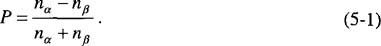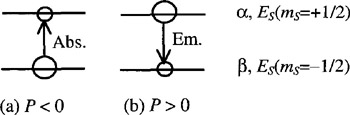Figure 5-1: ESR signals in (a) absorption and (b) in emission.

The population of the i-th level is denoted by n i( i = ? or ?), which is represented by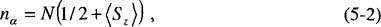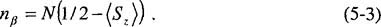In Eqs. (5-2) and (5-3), N is the total number of electron spins and < S z> is the net z-component of the spins. Eqs. (5-2) and (5-3) are reasonable because n a ( n ?) becomes N or 0 (0 or N) when < S z> is 1/2 or ?1/2. From Eqs. (5-1) (5-3), P can be given as follows: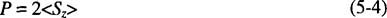Problem 5-1 Calculate the P values in the thermal equilibrium ( P eq) at room temperature (20 C) for the ESR transitions of an electron at the X-, K-, and Q-bands (9, 25, and 35 GHz). CIDEP is observed for the case of P < P eq (enhanced absorption) or P > 0 (emission) as shown in Fig. 5-1.